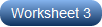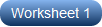# Decimal and Hexadecimal Conversion Worksheets

Don’t you wish your high school students were head and shoulders above their peers in converting between decimal and hexadecimal numbers? Page through our printable worksheets, and ace it with oodles of practice. The decimal number system, also called the base-10 number system, has 10 symbols from 0 to 16. The hexadecimal number system uses 16 different digits, including digits and letters. The pdf tasks here range from converting decimal to hex and vice versa to MCQs to more. Grab one of the worksheets for free!

Be a master of converting decimal numbers to hexadecimal numbers with our printable worksheets. Divide the number by 16; continue to divide until you get zero as the quotient. Also, replace the remainders with the equivalent base 16 values.Make progress in your learning by transforming the hexadecimals to decimals with this set of pdfs curated by experts. Simply multiply each digit with the decreasing power of 16 to arrive at the answer.Convert between Decimal and Hexadecimal - MCQ

Test your comprehension of conversion between decimal and hexadecimal numbers with these exercises in MCQ format. Read each question carefully and choose the correct answer by converting between the two number systems.